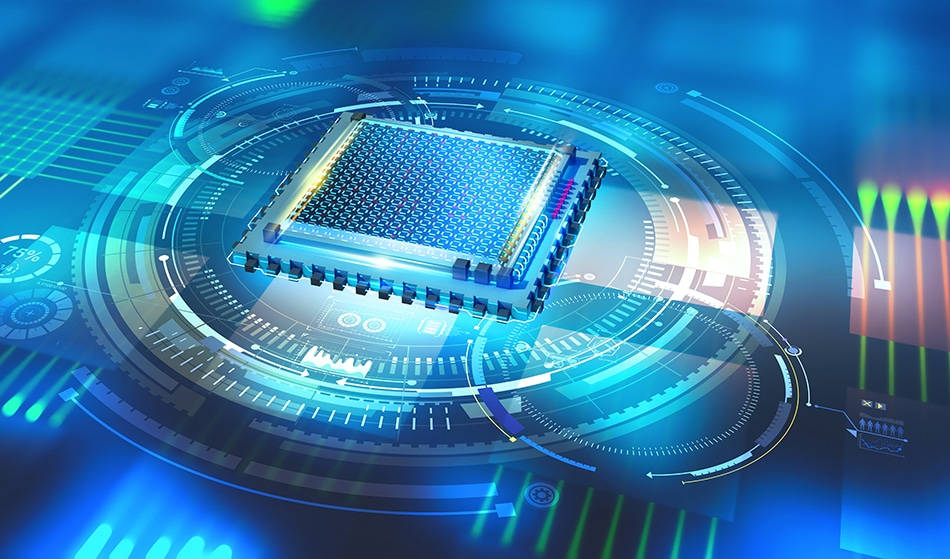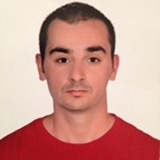# The Elements of Quantum ComputingYurchanka Siarhei / Shutterstock

Quantum computing is the study of processing tasks based on a computational model which uses quantum mechanical phenomena such as entanglement and superposition. Nobel Prize-winning physicist Richard Feynman discussed a quantum computer which could simulate physics in ways that a classical machine could not and his study can be regarded as the beginning of quantum computing.

Scientists now see quantum computing as an emerging field of science that could radically reshape the world. Therefore, it is important to understand that quantum computers have a computational mechanism that is radically different from our well-known classical computers.

## Bits vs Qubits

Classical computers process data in bits with states either |0⟩ or |1⟩. However, classical information theory is also built upon the concept of bit which is the smallest basic unit of information. Instead of bit, quantum computers encode data in a series of quantum bits, or qubits. The difference between bit and qubit is that the qubit is superposition of both |0⟩ and |1⟩.

|Ψ⟩ = α |0⟩ + β |1⟩

where α and β are complex numbers, and as a result we arrive at something that has no classical analog. If we measure the state of a bit, the outcome would be 1 or 0. In other words, a bit must be either one state or the other, similar to a flipped coin. When we measure qubit, the outcome ıs equally 1 or 0. Therefore, result of our measurement is completely uncertain, we get 0 with probability |α |2 or 1 with probability with |β|2. Furthermore, the use of entanglement between qubits allows for successful operation models such as quantum teleportation and superdense coding. As a result, quantum entanglement is a key concept of quantum computation. While the quantum mechanical concept of a qubit is difficult to grasp, their existence has been validated by experiments.

## Quantum Gates

If we want to construct a quantum computer we need a new kind of circuit which is a sequence of quantum gates. In classical computers, Boolean algebra is used to construct logic gates and computer circuits.  Therefore, to construct circuits for a quantum computer we require a new kind of gate. Simply, quantum gates are a quantum mechanical generalization of classical logic gates. The only difference is that the input and output states of quantum gates are superposition of both states |0⟩  and |1⟩. In other words, the eigenstates of quantum gates are a mixture of quantum states, instead of just one. To provide a better understanding of the action of a quantum gate, consider a NOT gate which switches state |0⟩ to state |1⟩.

α |0⟩ + β |1⟩ → α |1⟩ + β |0⟩

The superposition allows a quantum computer to hold more information up to two bits.

## Quantum Algorithms

A simplistic description of a quantum algorithm is an algorithm which gives exponential speedup over classical algorithms to solve a computational model. The distinction between quantum and classical algorithms is that the quantum algorithm inputs a superposition of all possible inputs and then runs on superposition, instead of performing a algorithm on one input like a classical algorithm. Consequently, to solve concrete problems using a quantum computer we require a quantum algorithm. Some examples of quantum algorithms are listed below.

• Shor’s Algorithm
• Grover’s Algorithm
• HS Algorithm
• Quantum walk

## Future of Quantum Computing

Currently, fundamentals of quantum computing and quantum information are well established. Furthermore, many researchers have developed different versions of computational models and plenty of quantum algorithms have been proposed, such as Shock’s algorithm for integer factoring problems and Grove’s algorithm for searching unstructured databases. However, the application of quantum computing to concrete problems is still at its infancy and some difficulties have been encountered in the development of quantum computers. The most important problem is that the hardware requirements of quantum computers include qubits. In conclusion, we cannot handle to problems of quantum computation yet, but scientists are confident that, like classical computers at the turn of this century, quantum computers will radically reshape our world in the near future.

## Sources

• Deutsch, D.: Quantum computational networks. Proc. of the Royal Society A 425, 73–90 (1989)
• Feynman, R.: Simulating physics with computers. International Journal of Theoretical Physics 21, 467–488 (1982)
• Feynman, R.: Quantum mechanical computer. Optics News 11, 11–20 (1985)
• Nielsen M, Chuang I, Quantum Computation and Quantum Information

Disclaimer: The views expressed here are those of the author expressed in their private capacity and do not necessarily represent the views of AZoM.com Limited T/A AZoNetwork the owner and operator of this website. This disclaimer forms part of the Terms and conditions of use of this website.Written by

### Kazim Gokhan Atman

Kazim Gokhan Atman is a Ph.D. student in mathematical physics. He is mainly focused on fractional calculus and possible violation of locality at a small distance in Quantum Field Theory.

## Citations

• APA

Atman, Kazim. (2019, November 20). The Elements of Quantum Computing. AZoQuantum. Retrieved on October 26, 2020 from https://www.azoquantum.com/Article.aspx?ArticleID=133.

• MLA

Atman, Kazim. "The Elements of Quantum Computing". AZoQuantum. 26 October 2020. <https://www.azoquantum.com/Article.aspx?ArticleID=133>.

• Chicago

Atman, Kazim. "The Elements of Quantum Computing". AZoQuantum. https://www.azoquantum.com/Article.aspx?ArticleID=133. (accessed October 26, 2020).

• Harvard

Atman, Kazim. 2019. The Elements of Quantum Computing. AZoQuantum, viewed 26 October 2020, https://www.azoquantum.com/Article.aspx?ArticleID=133.

## Tell Us What You Think